# Introduction

Graphs are a generalization of trees. Like trees, graphs have nodes and edges. (The nodes are sometimes called vertices, and the edges are sometimes called arcs.) However, graphs are more general than trees: In a graph, a node can have any number of incoming edges (in a tree, the root node cannot have any incoming edges, and the other nodes can only have one incoming edge). Every tree is a graph, but not every graph is a tree.

There are two kinds of graphs, directed and undirected: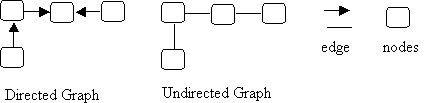Note that in a directed graph, the edges are arrows (are directed from one node to another) while in the undirected graph the edges are plain lines (they have no direction). In a directed graph, you can only go from node to node following the direction of the arrows, while in an undirected graph, you can go either way along an edge. This means that in a directed graph it is possible to reach a "dead end" (to get to a node from which you cannot leave).

# Terminology

Here are two example graphs (one directed and one undirected) and the terminology to describe them.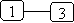In the directed graph, there is an edge from node 2 to node 1; therefore:

• The two nodes are adjacent (they are neighbors).
• Node 2 is a predecessor of node 1.
• Node 1 is a successor of node 2.
• The source of the edge is node 2, and the target of the edge is node 1.

In the undirected graph, there is an edge between node 1 and node 3; therefore:

• Nodes 1 and 3 are adjacent (they are neighbors).

Now consider the following (directed) graph: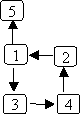In this graph, there is a path from node 2 to node 5: 2→1→5. There is a path from node 1 to node 2: 1→3→4→2. There is also a path from node 1 back to itself: 1→3→4→2→1. The first two paths are acyclic paths: no node is repeated; the last path is a cyclic path, because node 1 occurs twice.

Note that the layout of the graph is arbitrary -- the important thing is which nodes are connected to which other nodes. So, for example, the following graph is the same as the one given above, it's just been drawn differently: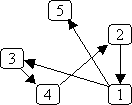Also note that an edge can connect a node to itself; for example: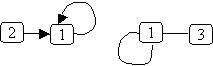## Some special kinds of graphs

• A directed graph that has no cyclic paths (that contains no cycles) is called a DAG (a Directed Acyclic Graph).

• An undirected graph that has an edge between every pair of nodes is called a complete graph. Here's an example:A directed graph can also be a complete graph; in that case, there must be an edge from every node to every other node.

• A graph that has values associated with its edges is called a weighted graph. The graph can be either directed or undirected. The weights can represent things like:
• The cost of traversing the edge.
• The length of the edge.
• The time needed to traverse the edge.
Here's an example of a weighted, directed graph: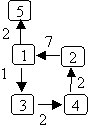• A graph is connected if, treating all edges as being undirected, there is a path from every node to every other node. For example:TEST YOURSELF #1

For each of the following graphs, say whether it is:

• connected or not connected
• complete or not complete
If the graph is a directed graph, also say whether it is cyclic or acyclic.# Uses for Graphs

In general, the nodes of a graph represent objects and the edges represent relationships. Here are some examples:

• Flights between cities. The nodes represent the cities, and there is an edge j → k iff there is a flight from city j to city k. This graph could be a weighted graph, using the weights to represent the distance, the flight time, or the cost.
• Interdependent tasks to be done. The nodes represent the tasks, and there is an edge j → k iff task j must be completed before task k. For example, we can use a graph to represent what must be done to finish a CS major, with the nodes representing the courses to be taken, and the edges representing prerequisites. Here's a partial example:• Flow charts (also known as control-flow graphs). The nodes represent the statements and conditions in a program, and the edges represent the flow of control.
• State transition diagrams. The nodes represent states, and the edges represent legal moves from state to state. For example, we could use a graph to represent legal moves in a game of tic-tac-toe. Here's a small part of that graph: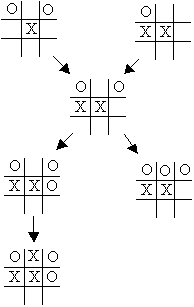The reason graphs are good representations in cases like those described above is that there are many standard graph algorithms (operations on graphs) that can be used to answer useful questions like:
• What is the cheapest way to fly from Madison to Saskatoon?
• Which CS classes must I take before I can take cs640?
• Is it possible for variable k to be used before being initialized?
• Can I win a game of tic-tac-toe starting from the current position?

# Representing Graphs

In a tree, all nodes can be reached from the root node, so a tree can be represented using two classes: a Treenode class (used to represent each individual node), and a Tree class that contains a pointer to the root node. Some graphs have a similar property; i.e., there is a special "root" node from which all other nodes are reachable (control-flow graphs often have this property). In that case, a graph can also be represented using a Graphnode class for the individual nodes, and a Graph class that contains a pointer to the root node. However, if there is no root node, then the Graph class needs to use some other data structure to keep track of the nodes in the graph. There are many possibilities: an array, a List, or a Set of Graphnodes could be used.

The Graphnodes will contain whatever data is stored in a node (e.g., the name of a city, the name of a CS class, the statement represented by a control-flow graph node). The nodes will also contain pointers to their successors (stored e.g., in an array, a List, or a Set).

Here's one reasonable pair of (incomplete) class definitions for directed graphs, using ArrayLists to store the nodes in the graph and the successors of each node:

```class Graphnode {
// *** fields ***
private Object data;
private ArrayList successors;

// *** methods ***
...
}

class Graph {
// *** fields ***
private ArrayList nodes;       // each item in the list will be a Graphnode

// *** methods ***
...
}
```

TEST YOURSELF #2

Suppose we have a weighted graph (one in which each edge has an associated value). How could the class definitions given above be extended to store the edge weights?

# Graph Operations

As discussed above, graphs are often a good representation for problems involving objects and their relationships because there are standard graph operations that can be used to answer useful questions about those relationships. Here we discuss two such operations: depth-first search and breadth-first search, and some of their applications.

Both depth-first and breadth-first search are "orderly" ways to traverse the nodes and edges of a graph that are reachable from some starting node. The main difference between depth-first and breadth-first search is the order in which nodes are visited. Of course, since in general not all nodes are reachable from all other nodes, the choice of the starting node determines which nodes and edges will be traversed (either by depth-first or breadth-first search).

## Depth-first Search

Depth-first search can be used to answer many questions about a graph:
• is it connected?
• is there a path from node j to node k?
• does it contain a cycle?
• what nodes are reachable from node j?
• can the nodes be ordered so that for every node j, j comes before all of its successors in the ordering?

The basic idea of a depth-first search is to start at some node n, and then to follow an edge out of n, then another edge out, etc, getting as far away from n as possible before visiting any more of n's successors. To prevent infinite loops in graphs with cycles, we must keep track of which nodes have been visited. Here is the basic algorithm for a depth-first search from node n, starting with all nodes marked "unvisited":

1. mark n "visited"
2. recursively do a depth-first search from each of n's unvisited successors
Information about which nodes have been visited can be kept in the nodes themselves (e.g., using a boolean field) or, if the nodes are numbered from 1 to N, the "visited" information can be stored in an auxiliary array of booleans of size N. Below is code for depth-first search, assuming that visited information is in a node field named "visited", and that each node's successors are in a List named "successors", and that the Graphnode class provides the usual get/set methods to access its fields. Note that this basic depth-first search doesn't actually do anything except mark nodes as having been visited. We'll see in the next section how to use variations on this code to do useful things.
```static void dfs (Graphnode n) {
n.setVisited( true );
Iterator it = n.getSuccessors().iterator();
while (it.hasNext()) {
Graphnode m = (Graphnode)it.next();
if (! m.getVisited()) dfs(m);
}
}
```
Here's a picture that illustrates the dfs method. In this example, node numbers are used to denote the nodes themselves (i.e., the call dfs(0) really means that the dfs method is called with a pointer to the node labeled 0). Two different colors are used to indicate the node currently being visited and the previously visited node.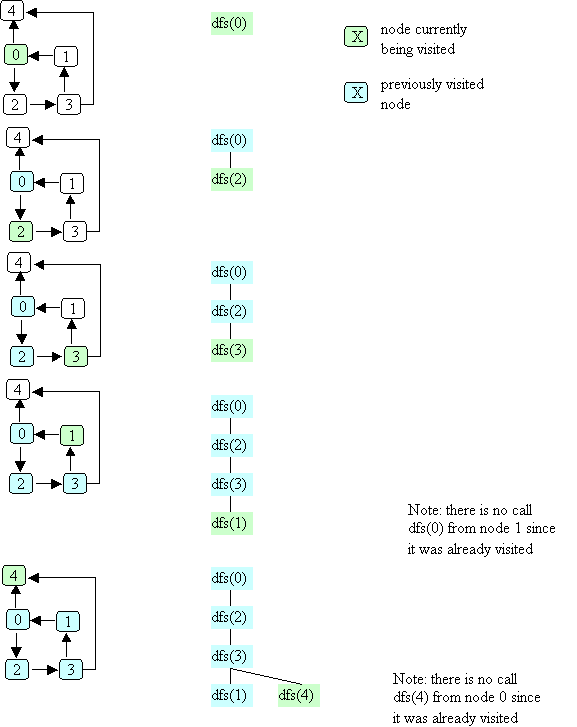Note that in the example illustrated above, the order in which the nodes are visited is: 0, 2, 3, 1, 4. Another possible order (if node 4 were the first successor of node 0) is: 0, 4, 2, 3, 1.

To analyze the time required for depth-first search, note that one call is made to dfs for each node that is reachable from the start node. Each call looks at all successors of the current node, so the time is O(# reachable nodes + total # of outgoing edges from those nodes). In the worst case, this is all nodes and all edges, so the worst-case time is O(N + E), where N is the number of nodes in the graph, and E is the number of edges in the graph.

TEST YOURSELF #3

Assume that you start with all nodes "unvisited", and you do a depth-first search. Write a (Graph) method that sets all nodes back to "unvisited".

### Uses for Depth-First Search

Recall that at the beginning of this section we said that depth-first search can be used to answers questions about a graph such as:

1. is it connected?
2. is there a path from node j to node k?
3. does it contain a cycle?
4. what nodes are reachable from node j?
5. can the nodes be ordered so that for every node j, j comes before all of its successors in the ordering?
Questions 2, 3 and 5 are discussed; the others are left as exercises.

#### Path Detection

The first question we will consider is: is there a path from node j to node k? This question might be useful, for example:

• When the graph represents airline routes, and we want to ask "Can I fly from Madison to London (maybe w/ some connections)?", or
• when the graph represent CS course prerequisites, and we want to ask "Is CS367 a (transitive) prerequisite for CS640?"
To answer the question, do the following:
• step 1: mark all nodes "not visited"
• step 2: dfs(j)
• step 3: there is a path from j to k iff k is marked "visited"

#### Cycle Detection

There are two variations that might be interesting:
1. does a graph contain a cycle?
2. is there a cyclic path starting from node j?

Consider the example given above to illustrate depth-first search. There is a cycle in that graph starting from node 0. Is there something that happens during the depth-first search that indicates the presence of that cycle?? Note that during dfs(1), 0 is a successor of 1, but is already visited. But that isn't quite enough to say that there's a cycle, because during dfs(3), node 4 is a successor of 3 that has already been visited, but there is no cycle starting from node 4.

What's the difference? The answer is that when node 0 is considered as a successor of node 1, the call dfs(0) is still "active" (i.e., its activation record is still on the stack); however, when node 4 is considered as a successor of node 3, the call dfs(4) has already finished. How can we tell the difference?? The answer is to keep track of when a node is "inProgress" (as well as whether it has been visited or not). We can do this by using a "mark" field with three possible values:

1. unvisited
2. inProgress
3. done
instead of the boolean "visited" field we've been using. Initially, all nodes are marked "unvisited". When the dfs method is first called for node n, it is marked "inProgress". Once all of its successors have been processed, it is marked "done". There is a cyclic path reachable from node n iff some node's successor is found to be marked "inProgress" during dfs(n).

Here's the code for cycle detection:

```static boolean hasCycle(Graphnode n) {
n.setMark( inProgress );
Iterator it = n.getSuccessors().iterator();
while(it.hasNext()) {
Graphnode m = it.next();
if (m.getMark() == inProgress) return true;
if (m.getMark() != done) {
if (hasCycle(m)) return true;
}
}
n.setMark( done );
return false;
}
```
Note that if we want to know whether a graph contains a cycle anywhere (not just one that is reachable from node n) we might have to call hasCycle at the "top-level" more than once. Here's a pseudo-code version of a method of the Graph class that returns true iff there is a cycle somewhere in the graph:
```public boolean graphHasCycle() {
mark all nodes unvisited;
for each node k in the graph {
if (node k is marked unvisited) {
if (hasCycle(k)) return true;
}
}
return false;
}
```

#### Topological Numbering

Think again about the graph that represents course prerequisites. As long as there are no cycles in the graph there is at least one order in which to take courses, such that all prereqs are satisfied; i.e., so that for every course, all prerequisites are taken before the course itself is taken. (Note that is is reasonable to assume that there are no cycles in a graph that represents course prerequisites, because a cycle would mean that a course was a prerequisite for itself!)

Topological numbering can be used to find the order in which to take the classes (so that all prereqs are satisfied first). The goal is to assign numbers to nodes so that for every edge j → k, the number assigned to j is less than the number assigned to k. A topological numbering of the prerequisites graph would tell you one legal order in which to take the CS courses. For example:To find a topological numbering, we use a variation of depth-first search. The intuition is as follows: As long as there are no cycles in the graph, there must be at least one node with no outgoing edges:

• The last number (N) can be given to any such node (310, 577, or 640 in our example).
• Once all of a node's successors have numbers, the node itself can get the next smallest number.
These 2 situations correspond to the point in method hasCycle where node n is marked "done" (when it has no more unvisited successors). We just need to keep track of the current number. Below is a method that, given a node n and a number num, assigns topological numbers to all unvisited nodes reachable from n, starting with num and working down. Note that before calling this method for the first time, all nodes should be marked "unvisited", and that the initial call should pass N (the number of nodes in the graph) as the 2nd parameter.
```static int topNum (Graphnode n, int num) throws CycleException {
n.setMark( inProgress );
Iterator it = n.getSuccessors().iterator();
while (it.hasNext()) {
Graphnode m = it.next();
if (m.getMark() == inProgress) {
// no topological ordering for a cyclic graph!
throw new CycleException();
}
if (m.getMark() != done) num = topNum(k, num);
}
// here when n has no more successors
n.setMark( done );
n.setNumber( num );
return num-1;
}
```
As was the case for cycle detection, we might need several "top-level" calls to number all nodes in a graph.

TEST YOURSELF #4

Question 1: Give two different topological numberings for the following graph.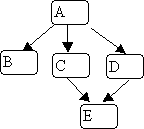Question 2: The topNum method given above only assigns numbers to the nodes reachable from node n. Write pseudo code for method numberGraph, similar to the code given for method graphHasCycle above, that assigns topological numbers to all nodes in a graph.

Question 3: Write a Graph method isConnected, that returns true iff the graph is connected. Assume that every node has a list of its predecessors as well as a list of its successors.

Breadth-first search provides another "orderly" way to visit (part of) a graph. The basic idea is to visit all nodes at the same distance from the start node before visiting farther-away nodes. Like depth-first search, breadth-first search can be used to find all nodes reachable from the start node. It can also be used to find the shortest path between two nodes in an unweighted graph.

Breadth-first search uses a queue rather than recursion (which actually uses a stack); the queue holds "nodes to be visited". If the graph is a tree, breadth-first search gives you a level-order traversal. Here's the pseudo code:

```static void bfs (Graphnode n) {
Queue Q = new Queue();

n.setVisited( true );
Q.enqueue( n );
while (! Q.empty())){
Graphnode current = (Graphnode)Q.dequeue();
Iterator it = current.getSuccessors().iterator();
while (it.hasNext()) {
Graphnode k = (Graphnode)it.next();
if (! k.getVisited()){
k.setVisited( true );
Q.enqueue( k );
} // end if k not visited
} // end for every successor k
} // end while Q not empty
}
```
Here's the same example graph we used for depth-first search, and an illustration of breadth-first search, starting with node 0: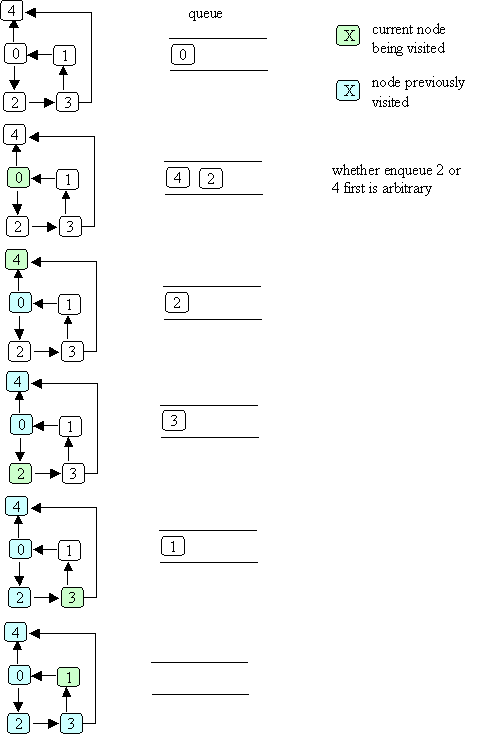The order in which nodes are "visited" as a result of bfs(0) is: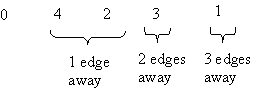As with depth-first search, all nodes marked "visited" are reachable from the start node, but nodes are visited in a different order than they would be using depth-first search.

We can use a variation of bfs to find the shortest distance (the length of the shortest path) to each reachable node:

• add a "distance" field to each node
• when bfs is called with node n, set n's distance to zero
• when a node k is about to be enqueued, set k's distance to the distance of the current node (the one that was just dequeued) + 1

This technique only works in unweighted graphs (i.e., in graphs in which all edges are assumed to have length 1). An interesting problem is how to find shortest paths in a weighted graph; i.e., given a "start" node n, to find, for each other node m, the path from n to m for which the sum of the weights on the edges is minimal (assuming that no edge has a negative weight). For example, in the following graph, nodes represent cities, edges represent highways, and the weights on the edges represent distances (the length of the highway between the two cities). Breadth-first search can only tell you which route from Madison to Green Bay goes through the fewest other cities; it cannot tell you which route is the shortest.A clever algorithm that can be used to solve this problem (to find shortest paths in a weighted graph with non-negative edge weights) has been defined by Edsgar Dijkstra (and so is called "Dijkstra's algorithm"). The worst-case running time of the algorithm is O(E log N), where E is the number of edges and N is the number of nodes. You can find a description of the algorithm in most data structures or algorithms textbooks; you are not responsible for understanding it for this class.

# SUMMARY

• A graph is a set of nodes and a set of edges. `
• There are two kinds of graphs: directed and undirected.
• High-level operations include:
• depth-first search, which can be done on the entire graph (e.g., to find cycles or to produce a topological ordering), or on part of a graph (e.g., to determine which nodes are reachable from a given node)
• breadth-first search, which can also be used to determine reachability, and can be used to find shortest paths in unweighted graphs
• Dijkstra's algorithm, which finds shortest paths in weighted graphs.